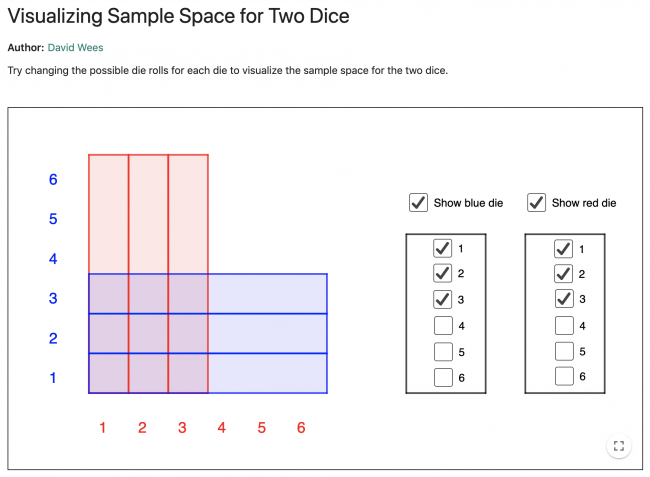# Visualizing Sample Space for Two Dice

Preview:This activity lets students visualize the sample space for two different numbered dice.

Technology
Big Idea:
Grade 4: Analyzing and interpreting experiments in data probability develops an understanding of chance.
Content:
probability experiments: predicting single outcomes (e.g., when you spin using one spinner and it lands on a single colour)
Curricular Competency:
Reasoning and analyzing: Use reasoning to explore and make connections
Reasoning and analyzing: Develop mental math strategies and abilities to make sense of quantities
Reasoning and analyzing: Use technology to explore mathematics
Understanding and solving: Visualize to explore mathematical concepts
Communicating and representing: Use mathematical vocabulary and language to contribute to mathematical discussions